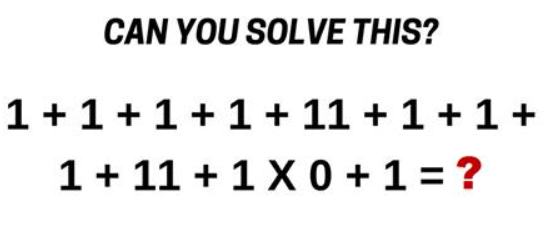﻿ Practice with Order of Operations
PRACTICE WITH ORDER OF OPERATIONS
by Dr. Carol JVF Burns (website creator)
Follow along with the highlighted text while you listen!
• PRACTICE (online exercises and printable worksheets)
Want more details, more exercises?

The order that operations are to be performed (when not clearly identified)
is summarized with the following memory device:

Please   Excuse   My   Dear   Aunt   Sally    (PEMDAS)

• Do things inside Parentheses first (using PEMDAS, if needed, inside the parentheses).
• Then do all Exponents, in order as they occur, going from left to right.
• Then do all Multiplications/Divisions (they have equal weight) in order as they occur, going from left to right.
• Finally, do all Additions/Subtractions (they have equal weight) in order as they occur, going from left to right.

In September 2017, this problem was floating around the web:Here's the solution, with correct order of operations: \begin{alignat}{2} &\cssId{s19}{1 + 1 + 1 + 1 + 11 + 1 + 1 + 1 + 11 + 1\times 0 + 1}&& \cr &\qquad\cssId{s20}{= 1 + 1 + 1 + 1 + 11 + 1 + 1 + 1 + 11 + (1\times 0) + 1} &\qquad&\cssId{s21}{\text{(the multiplication gets done first)}} \cr &\qquad\cssId{s22}{= 1 + 1 + 1 + 1 + 11 + 1 + 1 + 1 + 11 + 0 + 1}\cr &\qquad\cssId{s23}{= 30} \end{alignat} If this doesn't make sense to you, try the following mental exercise on a shorter (but similar) problem: $1 + 2 \times 0 + 3$
• Replace each plus sign with an (equally-weak) person.
• Replace the multiplication sign with a strong person.
Why? Multiplication is ‘stronger than’ addition!
And this makes perfectly good sense, since multiplication is ‘super-addition’: for example, $\,5\times 2 = 2 + 2 + 2 + 2 + 2\,$.
• The first (left-most) weak guy is trying to pull together the $\,1\,$ and the $\,2\,$, to add them.
• The middle (strong) guy is trying to pull together the $\,2\,$ and the $\,0\,$, to multiply them.
• The right-most weak guy is trying to pull together the $\,0\,$ and the $\,3\,$, to add them.
• Who wins? Clearly, the strong guy!

1203
$$\cssId{s37}{1 + 2 \times 0 + 3} \ \ \cssId{s38}{=\ \ 1 + \overbrace{(2\times 0)}^{\text{strong guy wins}} + 3} \ \ \cssId{s39}{=\ \ 1 + 0 + 3} \ \ \cssId{s40}{=\ \ 4}$$
MORE EXAMPLES:
$\cssId{s42}{-1 + 3\times 5 - 2} \cssId{s43}{= -1 + (3\times 5) - 2} \cssId{s44}{= 12}$
$\cssId{s45}{2 - 10\div 5 + 3} \cssId{s46}{= 2 - \frac{10}{5} + 3} \cssId{s47}{= 3}$
Master the ideas from this section

When you're done practicing, move on to the optional section:
Laurel, Yanny, Cookies, Bananas, and Clocks

Or, move on to:
Taking PEMDAS Too Literally: Don't Make This Mistake!

Feel free to use a pencil and scrap paper to work these problems.
However, do not use your calculator!

Simplify: